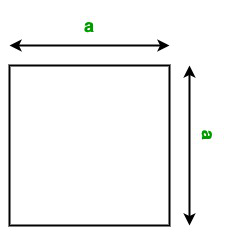Open in App
Not now

• Difficulty Level : Medium
• Last Updated : 08 Sep, 2022

A square is a simple flat shape in a plane, defined by four points at the four corners. It has four sides with equal length and four corners with right angles.

Method Overloading: Method overloading allows different methods to have the same name, but different signatures where the signature can differ by the number of input parameters or type of input parameters, or both.

#### Area of the Square

The area of the square is the square of its sides. We can simply calculate the area of the square using the following formula:Formula:

Area of the Square: A = a2

Here, a is the side of the square.

Below is the implementation of the above approach:

Example 1:

Showing the method overloading of Area() function which has different parameters of  square sides of different data-type( float , int , double)

## Java

 `// Java program to find the area of``// the square using Method Overloading``// of parameters with different datatype``// of sides` `import` `java.io.*;` `class` `Square {` `    ``// Overloaded Area() function to``    ``// calculate the area of the square``    ``// It takes one double parameter``    ``void` `Area(``double` `side)``    ``{``        ``System.out.println(``"Area of the Square: "``                           ``+ side * side);``    ``}` `    ``// Overloaded Area() function to``    ``// calculate the area of the square``    ``// It takes one float parameter``    ``void` `Area(``float` `side)``    ``{``        ``System.out.println(``"Area of the Square: "``                           ``+ side * side);``    ``}``}` `class` `GFG {` `    ``// Driver code``    ``public` `static` `void` `main(String[] args)``    ``{` `        ``// Creating object of square class``        ``Square obj = ``new` `Square();` `        ``// Calling function``        ``obj.Area(``10``);``        ``obj.Area(``3.2``);``    ``}``}`

Output

```Area of the Square: 100.0
Area of the Square: 10.240000000000002```

Example 2:

Showing method overloading of Area() function which returns the area of different figures like( square, circle, rectangle)

## Java

 `// Java program to find the area of``// the multiple shapes``// using Method Overloading` `import` `java.io.*;` `class` `Shape {` `    ``static` `final` `double` `PI = Math.PI;` `    ``// Overloaded Area() function to``    ``// calculate the area of the square``    ``// It takes one float parameter``    ``void` `Area(``float` `a)``    ``{``        ``float` `A = a * a;``        ``System.out.println(``"Area of the Square: "` `+ A);``    ``}` `    ``// Overloaded Area() function to``    ``// calculate the area of the circle``    ``// It takes one double parameter``    ``void` `Area(``double` `a)``    ``{``        ``double` `A = PI * a * a;``        ``System.out.println(``"Area of the Circle: "` `+ A);``    ``}` `    ``// Overloaded Area() function to``    ``// calculate the area of the rectangle``    ``// It takes two int parameters``    ``void` `Area(``int` `a, ``int` `b)``    ``{``        ``int` `A = a * b;``        ``System.out.println(``"Area of the Rectangle: "` `+ A);``    ``}``}` `class` `GFG {` `    ``// Driver code``    ``public` `static` `void` `main(String[] args)``    ``{` `        ``// Creating object of Shape class``        ``Shape obj = ``new` `Shape();` `        ``// Calling function``        ``obj.Area(``10.5``);``        ``obj.Area(``3``);``        ``obj.Area(``5``, ``4``);``    ``}``}`

Output

```Area of the Circle: 346.36059005827474
Area of the Square: 9.0
Area of the Rectangle: 20```

Time complexity: O(1) because it is doing constant operations

Auxiliary space: O(1)

My Personal Notes arrow_drop_up